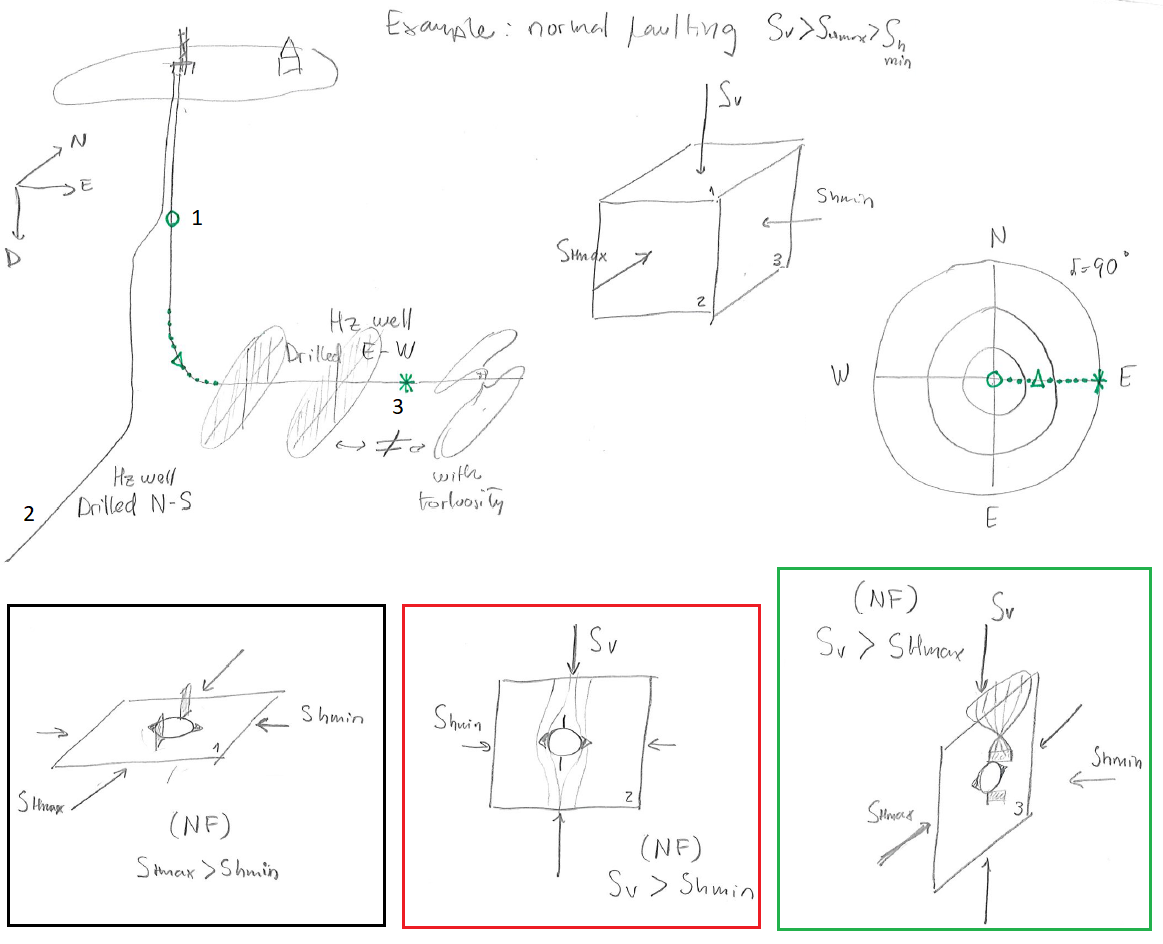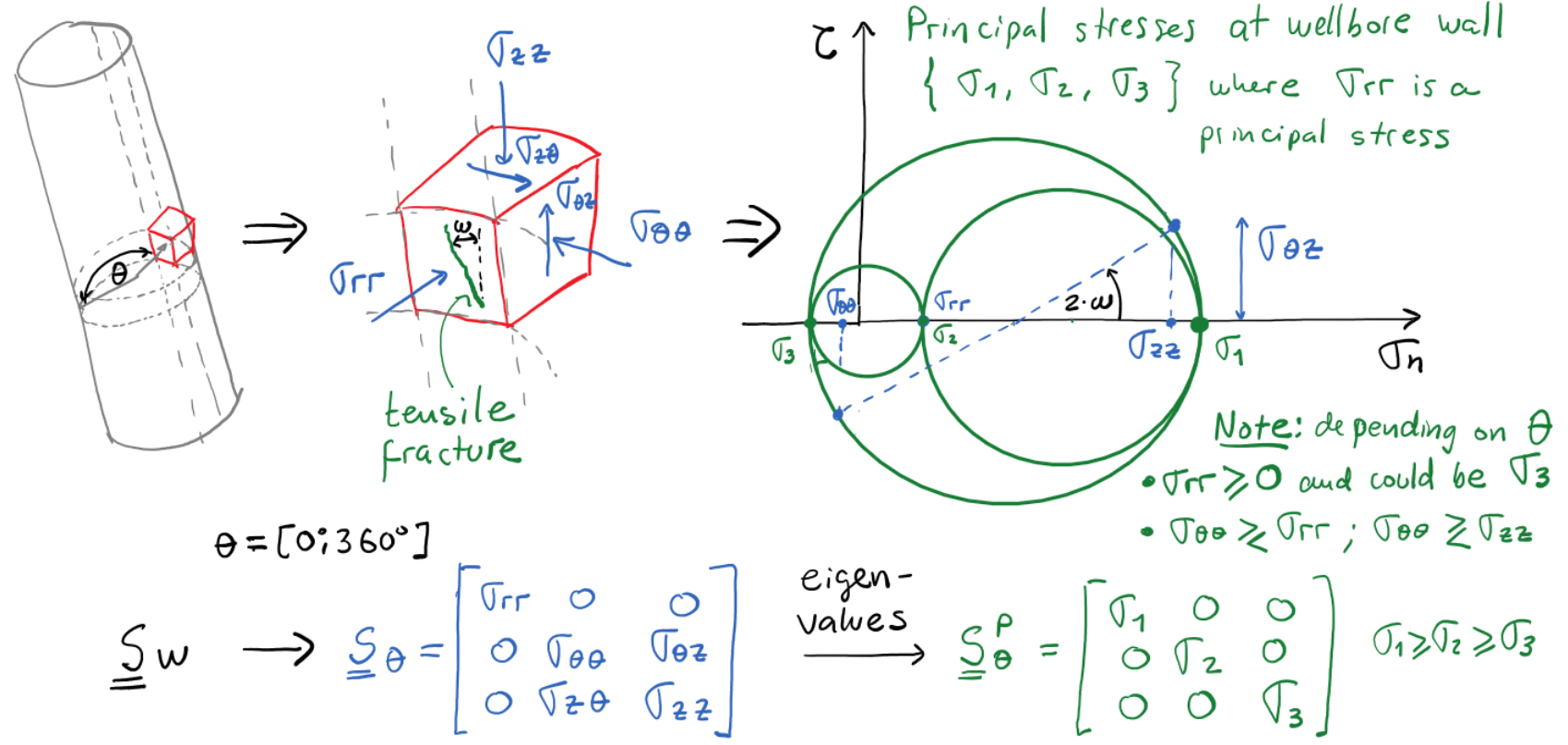Subsections

# 6.6 Mechanical stability of deviated wellbores

The following subsections present a guide for calculating stresses at the wall of deviated wellbores and identifying stress magnitudes and locations for shear failure (breakouts) and tensile fractures.

## 6.6.1 Wellbore orientation

At any point along the trajectory of a deviated wellbore, the tangent orientation permits defining wellbore azimuthand deviation(Fig. 6.19). Azimuthis the angle between the projection of the trajectory on a horizontal plane and the North. Deviationis the angle between a vertical line and the trajectory line at the point of consideration. These two variables can be plotted in a half-hemisphere projection plot (stereonet). Notice that a point in this plot represents just one point along a wellbore trajectory. Fig. 6.19 shows an example of the full trajectory of a wellbore.A given state of stress will result in different mud windows and locations of rock failure depending on the wellbore orientation. Breakouts and tensile fractures will depend on the stresses on the plane perpendicular to the wellbore. Figure 6.20 shows an example for normal faulting with generic stress magnitude values.PROBLEM 6.4: Consider a place where vertical stressis a principal stress and the maximum horizontal stress acts in E-W direction.

1. Find the planes with maximum stress anisotropy for normal faulting, strike-slip, and reverse faulting stress regimes.
2. Plot the orientation of wellbores in those planes of maximum stress anisotropy in a stereonet projection plot.
3. Where around the wellbore would breakouts and tensile fractures occur in each case?

SOLUTION
The solution below shows just one of the possible solutions of two horizontal wells.## 6.6.2 Calculation of stresses on deviated wellbores

Let us define a coordinate system for a point along the trajectory of a deviated wellbore. The first elementof the cartesian base goes from the center of a cross-section of the wellbore at a given depth to the deepest point around the cross-section (perpendicular to the axis). The second element of the basegoes from the center to the side on a horizontal plane. The third element of the basegoes along the direction of the wellbore.Based on the previous definition, it is possible to construct a transformation matrixthat links the geographical coordinate system and the wellbore coordinate system.(6.19)

Furthermore, the wellbore stresses can be calculated from the principal stress tensor according with:(6.20)

Whereandare the principal stress tensor and the corresponding change of coordinate matrix to the geographical coordinate system (Eq. 5.7). The tensoris composed by the following stresses:(6.21)

Stresses on the plane of the cross-section of the deviated wellbore at the wellbore walldepend on far-field stresses,,,,, and. The Kirsch equations require additional far field shear terms,, andin order to account for principal stresses not coinciding with the wellbore orientation. The solution of Kirsch equation for isotropic rock with far-field shear stresses is provided in Fig. 6.22.Solving for the local principal stresseson the wellbore wall permits checking for rock failure (tensile or shear). The local principal stresses may not be necessarily aligned with the wellbore axis leading to an angle(see Fig. 6.23). Because of such angle, tensile fractures in deviated wellbores can occur at an anglefrom the axis of the wellbore and appear as a series of short inclined (en-échelon) fractures instead of a long tensile fracture parallel to the wellbore axis as in Fig. 6.16.## 6.6.3 Breakout analysis for deviated wellbores

Consider a place subjected to strike-slip stress regime withoriented at an azimuth of 070with known values of principal stresses (Fig 6.24). The maximum stress anisotropy lies in a plane that containsand, a plane perpendicular to the axis of a vertical wellbore. Hence, maximum stress amplification at the wellbore wall will happen for a vertical wellbore. The minimum stress anisotropy lies in a plane that containsand, perpendicular to a horizontal wellbore drilled in direction of. Given a mud pressure and a fixed friction angle, we can calculate for a given wellbore orientation the stresses on the wellbore wall from equations in Fig. 6.23, and the required(using a shear failure criterion) to avert shear failure. The plots in Fig. 6.24 are examples of this calculation. The maximum value of requiredcorresponds to the wellbore direction with maximum stress anisotropy (vertical wellbore - red region), and the minimum value of requiredcorresponds to the wellbore direction with minimum stress anisotropy (horizontal wellbore with- blue region). Following the breakout concepts discussed before, we would expect breakouts at 160and 340of azimuth on the sides of a vertical wellbore. A horizontal wellbore drilled in the direction ofwould tend to develop breakouts on the top and bottom of the wellbore.PROBLEM 6.5: Consider a place with principal stressesMPa,MPa (at 070),MPa,MPa, andMPa. Calculate the required UCS using the Coulomb failure criterion (with) for all possible wellbore orientations. Plot results in a stereonet projection.

SOLUTIONPROBLEM 6.6: Consider a place with principal stressesMPa,MPa (at 070),MPa,MPa, andMPa. Calculate the required UCS using the Coulomb failure criterion (with) for all possible wellbore orientations. Plot results in a stereonet projection.

SOLUTION## 6.6.4 Tensile fractures analysis for deviated wellbores

The procedure to find tensile failure is equivalent to the one used for shear failure, but using a tensile strength failure criterion. For example, consider a place subjected to normal faulting stress regime withoriented at an azimuth of 070and known values of principal stresses (Fig. 6.25). The maximum stress anisotropy lies in a plane that containsand, perpendicular to a horizontal wellbore drilled in the direction of. For a given rock tensile strength, we can calculate the maximum mud pressure that the wellbore can bear without failing in tension. Fig. 6.25 shows an example of this calculation. The maximum possiblecorresponds to the wellbore direction with minimum stress anisotropy (blue region), and the minimum possiblecorresponds to the wellbore direction with maximum stress anisotropy (red region). For example, tensile fractures would tend to occur in the top and bottom of a horizontal wellbore drilled in the direction of.PROBLEM 6.7: Consider a place with principal stressesMPa,MPa (at 070),MPa, andMPa. Calculate the maximum wellbore pressureat the limit of tensile strength withMPa) for all possible wellbore orientations. Plot results in a stereonet projection.

SOLUTION
TBD

PROBLEM 6.8: Consider a place with principal stressesMPa,MPa (at 070),MPa, andMPa. Calculate the maximum wellbore pressureat the limit of tensile strength withMPa) for all possible wellbore orientations. Plot results in a stereonet projection.

SOLUTION
TBD